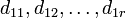# Degrees of irreducible representations of direct product are pairwise products of degrees of irreducible representations of direct factors

## Statement for two groups

### Over any field

Suppose$G_1$ and$G_2$ are finite groups. Suppose$K$ is a field. Suppose$d_{11},d_{12},\dots,d_{1r}$ are the degrees of irreducible representations (possibly with repetitions) of$G_1$ over$K$ and$d_{21},d_{22},\dots,d_{2s}$ are the degrees of irreducible representations of$G_2$.

Then, the degrees of irreducible representations of$G_1 \times G_2$ over$K$ are:$d_{11}d_{21}, d_{11}d_{22}, \dots,d_{11}d_{2s},d_{12}d_{21},d_{12}d_{22},\dots,d_{12}d_{2s},d_{1r}d_{21},\dots,d_{1r}d_{2s}$

### Over a splitting field

If we take$K$ to be a splitting field for both$G_1$ and$G_2$, then the above relates the degrees of irreducible representations for$G_1,G_2,G_1 \times G_2$ over a splitting field. For instance, we could take$K$ to be$\mathbb{C}$ or$\overline{\mathbb{Q}}$. Note that degrees of irreducible representations are the same for all splitting fields (as long as the characteristic does not divide the group order), so this relates universal lists of numbers for$G_1,G_2,G_1 \times G_2$.